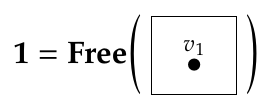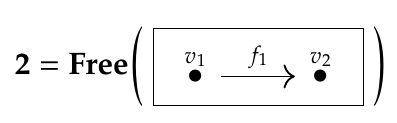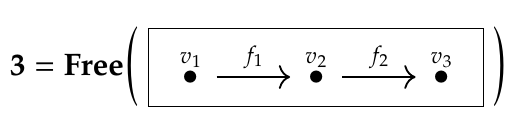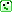#### Howdy, Stranger!

It looks like you're new here. If you want to get involved, click one of these buttons!

Options

# Lecture 49 - Chapter 3: Kan Extensions

edited February 18

Since adjoint functors are the deepest concept in the course so far, I'll need to keep explaining them before they sink in. There are a lot of technical issues we need to discuss, but you may want to study examples first. We started looking at some examples last time. We need to see more, and think about them harder.

But I can't resist getting to the punchline: the application to databases. I'm hoping that seeing the big picture will help you understand how all the pieces fit together.

In Lecture 41 we saw a trick for getting new databases from old.

Remember, a database schema is just a category $$\mathcal{C}$$. An database built using this schema is a functor

$$F : \mathcal{C} \to \mathbf{Set} .$$ If we also have a functor $$G: \mathcal{D} \to \mathcal{C}$$, we can take $$F$$ and compose it with $$G$$ to get a functor

$$F \circ G : \mathcal{D} \to \mathbf{Set} .$$ But this is just a database built using the schema $$\mathcal{D}$$!

So that's a pretty obvious trick for turning databases built using the schema $$\mathcal{C}$$ into databases built using the schema $$\mathcal{D}$$. But often we want to reverse this procedure, and turn databases built using $$\mathcal{D}$$ into databases built using $$\mathcal{C}$$.

There turn out to be two ways to reverse this procedure: one using a left adjoint, and one using a right adjoint. Remember, the point of adjoints is to reverse computations

Let me sketch the idea, which is really slick. There's actually a category whose objects are databases built using the schema $$\mathcal{C}$$, or in other words, functors $$F : \mathcal{C} \to \mathbf{Set}$$. It's the functor category

$$\mathbf{Set}^{\mathcal{C}} .$$ This is why I introduced functor categories in Lecture 45! Similarly, there's a functor category

$$\mathbf{Set}^{\mathcal{D}}$$ whose objects are databases built using the schema $$\mathcal{D}$$.

But we've just seen that composing with $$G: \mathcal{D} \to \mathcal{C}$$ takes functors $$F : \mathcal{C} \to \mathbf{Set}$$ and gives functors $$F \circ G : \mathcal{D} \to \mathbf{Set}$$. In fact - this takes some work to check - this gives a functor

$$\textrm{composing with } G : \mathbf{Set}^{\mathcal{C}} \to \mathbf{Set}^{\mathcal{D}} .$$ And here's the cool part: this functor always has both a left adjoint and a right adjoint! These are ways to turn databases built using $$\mathcal{D}$$ into databases built using $$\mathcal{C}$$.

These adjoints, by the way, are called Kan extensions. The left adjoint is called the left Kan extension along $$G$$, and it's denoted

$$\mathrm{Lan}_G : \mathbf{Set}^{\mathcal{D}} \to \mathbf{Set}^{\mathcal{C}}$$ where 'Lan' is a weird abbreviation of 'left Kan'. The right adjoint is called the right Kan extension along $$G$$, and it's denoted

$$\mathrm{Ran}_G : \mathbf{Set}^{\mathcal{D}} \to \mathbf{Set}^{\mathcal{C}}.$$ Okay, that's a lot of abstraction! Next time we need to look at some examples.

In fact you've already seen some examples, last time. You just need to see that they are examples.

I'll explain this next time. But just for those of you who like a challenge:

Puzzle 154. Which of the functors in the example and puzzles from last time last time are of the form

$$\textrm{composing with } G : \mathbf{Set}^{\mathcal{C}} \to \mathbf{Set}^{\mathcal{D}} ?$$ Which are left or right adjoints of functors of this form?

Okay, maybe this is too 'meta': it's a puzzle about puzzles! But if you're taking a course on category theory, maybe you like 'meta'.

To read other lectures go here.

• Options
1.
edited June 2018

But we've just seen that composing with $$G: \mathcal{D} \to \mathcal{C}$$ takes functors $$F : \mathcal{C} \to \mathbf{Set}$$ and gives functors $$F \circ G : \mathcal{D} \to \mathbf{Set}$$. In fact - this takes some work to check - this gives a functor $$\textrm{composing with } F : \mathbf{Set}^{\mathcal{C}} \to \mathbf{Set}^{\mathcal{D}} .$$

Shouldn't it be "$$\textrm{composing with } G$$"? After all this functor takes any functor $$X \in \mathbf{Set}^{\mathcal{C}}$$ to a functor in $$\mathbf{Set}^{\mathcal{D}}$$, not just the functor $$F$$.

Likewise, later, shouldn't we talk about "the left/right Kan extension along G" (of functors $$F \in \mathbf{Set}^{\mathcal{C}}$$)?

Comment Source:> But we've just seen that composing with \$$G: \mathcal{D} \to \mathcal{C}\$$ takes functors \$$F : \mathcal{C} \to \mathbf{Set} \$$ and gives functors \$$F \circ G : \mathcal{D} \to \mathbf{Set} \$$. In fact - this takes some work to check - this gives a functor >$\textrm{composing with } F : \mathbf{Set}^{\mathcal{C}} \to \mathbf{Set}^{\mathcal{D}} .$ Shouldn't it be "\$$\textrm{composing with } G \$$"? After all this functor takes *any* functor \$$X \in \mathbf{Set}^{\mathcal{C}} \$$ to a functor in \$$\mathbf{Set}^{\mathcal{D}} \$$, not just the functor \$$F\$$. Likewise, later, shouldn't we talk about "the left/right Kan extension along G" (of functors \$$F \in \mathbf{Set}^{\mathcal{C}} \$$)?
• Options
2.
edited June 2018

Valter: yes, I'm composing with and Kan extending along $$G$$. $$F$$ here is just a name for an arbitrary object in $$\mathbf{Set}^\mathcal{C}$$. I'll fix that.

Comment Source:Valter: yes, I'm composing with and Kan extending along \$$G\$$. \$$F\$$ here is just a name for an arbitrary object in \$$\mathbf{Set}^\mathcal{C}\$$. I'll fix that.
• Options
3.
edited June 2018

Just to help people think about the functors from the examples and puzzles, I'm making a list here:

• The unique functor $$F:\mathbf{Set}\to\mathbf{1}$$
• Its right adjoint $$G:\mathbf{1}\to\mathbf{Set}$$
• Its left adjoint $$L:\mathbf{1}\to\mathbf{Set}$$
• The functor $$F':\mathbf{Set}^2\to\mathbf{Set}$$ throwing away the second component.
• Its right adjoint(s) $$G':\mathbf{Set}\to\mathbf{Set}^2$$, if there are any (Puzzle 150).
• Its left adjoint(s) $$L':\mathbf{Set}\to\mathbf{Set}^2$$, if there are any (Puzzle 151).
• The functor $$\times:\mathbf{Set}^2\to\mathbf{Set}$$ taking the cartesian product of the two sets.
• Its right adjoint(s) $$R_\times: \mathbf{Set}\to\mathbf{Set}^2$$, if there are any (Puzzle 152).
• Its left adjoints(s) $$L_\times: \mathbf{Set}\to\mathbf{Set}^2$$, if there are any (Puzzle 153).
• The functor $$+:\mathbf{Set}^2\to\mathbf{Set}$$ taking the disjoint union of the two sets.
• Its right adjoint(s) $$R_+: \mathbf{Set}\to\mathbf{Set}^2$$, if there are any (Puzzle 152').
• Its left adjoints(s) $$L_+: \mathbf{Set}\to\mathbf{Set}^2$$, if there are any (Puzzle 153').

I feel like there's a prerequisite puzzle: Which of the categories $$\mathbf{1},\mathbf{Set},\mathbf{Set}^2$$ used above are of the form $$\mathbf{Set}^\mathcal{C}$$ for some category $$C$$? The answer is: if you're willing to relabel the objects, all of them: $$\mathbf{1}\cong \mathbf{Set}^\mathbf{0}$$, $$\mathbf{Set}\cong\mathbf{Set}^\mathbf{1}$$, and $$\mathbf{Set}^2\cong \mathbf{Set}^\mathbf{2}$$, where for $$n\in\{0,1,2\}$$ I'm using $$\mathbf{n}$$ to denote the category with $$n$$ objects and only identity morphisms.

Comment Source:Just to help people think about the functors from the examples and puzzles, I'm making a list here: - The unique functor \$$F:\mathbf{Set}\to\mathbf{1}\$$ - Its right adjoint \$$G:\mathbf{1}\to\mathbf{Set}\$$ - Its left adjoint \$$L:\mathbf{1}\to\mathbf{Set}\$$ - The functor \$$F':\mathbf{Set}^2\to\mathbf{Set}\$$ throwing away the second component. - Its right adjoint(s) \$$G':\mathbf{Set}\to\mathbf{Set}^2\$$, if there are any (Puzzle 150). - Its left adjoint(s) \$$L':\mathbf{Set}\to\mathbf{Set}^2\$$, if there are any (Puzzle 151). - The functor \$$\times:\mathbf{Set}^2\to\mathbf{Set}\$$ taking the cartesian product of the two sets. - Its right adjoint(s) \$$R_\times: \mathbf{Set}\to\mathbf{Set}^2\$$, if there are any (Puzzle 152). - Its left adjoints(s) \$$L_\times: \mathbf{Set}\to\mathbf{Set}^2\$$, if there are any (Puzzle 153). - The functor \$$+:\mathbf{Set}^2\to\mathbf{Set}\$$ taking the disjoint union of the two sets. - Its right adjoint(s) \$$R_+: \mathbf{Set}\to\mathbf{Set}^2\$$, if there are any (Puzzle 152'). - Its left adjoints(s) \$$L_+: \mathbf{Set}\to\mathbf{Set}^2\$$, if there are any (Puzzle 153'). I feel like there's a prerequisite puzzle: Which of the categories \$$\mathbf{1},\mathbf{Set},\mathbf{Set}^2\$$ used above are of the form \$$\mathbf{Set}^\mathcal{C}\$$ for some category \$$C\$$? The answer is: if you're willing to relabel the objects, all of them: \$$\mathbf{1}\cong \mathbf{Set}^\mathbf{0}\$$, \$$\mathbf{Set}\cong\mathbf{Set}^\mathbf{1}\$$, and \$$\mathbf{Set}^2\cong \mathbf{Set}^\mathbf{2}\$$, where for \$$n\in\\{0,1,2\\}\$$ I'm using \$$\mathbf{n}\$$ to denote the category with \$$n\$$ objects and only identity morphisms.
• Options
4.
edited June 2018

Owen - thanks for helping out! I get the feeling my recent lectures are starting to blow people away. Too many big new concepts. Maybe I should stop presenting brand new information for a week and just help people absorb all the concepts. But you just helped a lot.

Indeed, all the categories in Lecture 48 are of the form $$\mathbf{Set}^\mathcal{C}$$ for various choices of $$\mathcal{C}$$.

This is no coincidence: I did it because we're studying databases! A database is a functor $$F : \mathcal{C} \to \mathbf{Set}$$ for some category $$\mathcal{C}$$ called the 'database schema'... so a database is an object in $$\mathbf{Set}^\mathcal{C}$$.

I'm using $$\mathbf{n}$$ to denote the category with $$n$$ objects and only identity morphisms.

That's fine as long as we warn people what we mean. But folks should be careful: Fong and Spivak (and the rest of the world) use $$\mathbf{n}$$ to mean something else! I mentioned this in Lecture 44.and so on: for any natural number $$n$$, people define the category $$\mathbf{n}$$ to be the free category on a graph with nodes $$v_1, \dots, v_n$$ and edges $$f_i : v_i \to v_{i+1}$$ where $$1 \le i < n$$.

So, to avoid conflict, we could call the categories you're talking about $$\mathbf{0}, \mathbf{1}, \mathbf{1} + \mathbf{1}, \mathbf{1} + \mathbf{1} + \mathbf{1}$$, and so on.

This is literally true: someday we may learn about the coproduct of categories, and we'll learn that $$+$$ stands for coproduct, and that $$\mathbf{1} + \mathbf{1}$$ (for example) is the category with two objects and only identity morphisms.

Unfortunately this way of setting up notation makes $$\mathbf{1} + \mathbf{1}$$ different from $$\mathbf{2}$$, so undergrads listening into my category theory courses will think I'm crazy!Comment Source:Owen - thanks for helping out! I get the feeling my recent lectures are starting to blow people away. Too many big new concepts. Maybe I should stop presenting brand new information for a week and just help people absorb all the concepts. But you just helped a lot. Indeed, _all_ the categories in [Lecture 48](https://forum.azimuthproject.org/discussion/2264/lecture-48-chapter-3-adjoint-functors/p1) are of the form \$$\mathbf{Set}^\mathcal{C}\$$ for various choices of \$$\mathcal{C}\$$. This is no coincidence: I did it because we're studying databases! A database is a functor \$$F : \mathcal{C} \to \mathbf{Set}\$$ for some category \$$\mathcal{C}\$$ called the 'database schema'... so a database is an object in \$$\mathbf{Set}^\mathcal{C}\$$. > I'm using \$$\mathbf{n}\$$ to denote the category with \$$n\$$ objects and only identity morphisms. That's fine as long as we warn people what we mean. But folks should be careful: Fong and Spivak (and the rest of the world) use \$$\mathbf{n}\$$ to mean something else! I mentioned this in [Lecture 44](https://forum.azimuthproject.org/discussion/2247/lecture-44-chapter-3-categories-functors-and-natural-transformations/p1). <center><img src = "http://math.ucr.edu/home/baez/mathematical/7_sketches/category_1.png"></center> <center><img src = "http://math.ucr.edu/home/baez/mathematical/7_sketches/category_2.png"></center> <center><img src = "http://math.ucr.edu/home/baez/mathematical/7_sketches/category_3.png"></center> and so on: for any natural number \$$n\$$, people define the category \$$\mathbf{n}\$$ to be the free category on a graph with nodes \$$v_1, \dots, v_n\$$ and edges \$$f_i : v_i \to v_{i+1}\$$ where \$$1 \le i < n\$$. So, to avoid conflict, we could call the categories you're talking about \$$\mathbf{0}, \mathbf{1}, \mathbf{1} + \mathbf{1}, \mathbf{1} + \mathbf{1} + \mathbf{1} \$$, and so on. This is literally true: someday we may learn about the coproduct of categories, and we'll learn that \$$+\$$ stands for coproduct, and that \$$\mathbf{1} + \mathbf{1} \$$ (for example) is the category with two objects and only identity morphisms. Unfortunately this way of setting up notation makes \$$\mathbf{1} + \mathbf{1}\$$ different from \$$\mathbf{2}\$$, so undergrads listening into my category theory courses will think I'm crazy! <img src = "http://math.ucr.edu/home/baez/emoticons/confused_rolleyes.gif"> 
• Options
5.
edited June 2018

Why not just use $$\mathrm{Discr}(\mathbf{n})$$ for a discrete category on $$n$$ objects?

Then $$\mathbf{Set}^{\mathrm{Discr}(\mathbf{n})}$$ is a database, for every discrete database schema $$\mathrm{Discr}(\mathbf{n})$$.

Comment Source:Why not just use \$$\mathrm{Discr}(\mathbf{n})\$$ for a discrete category on \$$n\$$ objects? Then \$$\mathbf{Set}^{\mathrm{Discr}(\mathbf{n})}\$$ is a database, for every discrete database schema \$$\mathrm{Discr}(\mathbf{n})\$$.
• Options
6.

Okay, sounds good. I think people sometimes use $$\mathrm{Disc}(S)$$ for the discrete category on the set $$S$$, and people definitely use $$n$$ to mean the set $$\{0, \dots, n-1\}$$, so we could also call this category $$\mathrm{Disc}(n)$$... which is almost what you wrote!

Comment Source:Okay, sounds good. I think people sometimes use \$$\mathrm{Disc}(S)\$$ for the [discrete category](https://en.wikipedia.org/wiki/Discrete_category) on the set \$$S\$$, and people definitely use \$$n\$$ to mean the set \$$\\{0, \dots, n-1\\}\$$, so we could also call this category \$$\mathrm{Disc}(n)\$$... which is almost what you wrote!